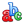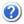Guest |Rus ||Figure 3. Experimental spectra of the H2O continuum in far infrared   range approximated by the model allowing for the contribution from the water dimers and the empirically calculated contribution from the line wings.The dots are the experimental data,the bold solid curve represents the model,the bold dashed curve,the thin dashed curve,and the thin solid curve are the contributions from the bound dimers,metastable dimers,and line wings, respectively. (a)The contribution from the line wings calculated by using Eqs. (8)–(10). A=14  for the left-hand  panel,and Δνwing=11 cm-1 in both cases. P=1 atm. T=296 K. (b) The contribution from the line wings calculated by using Eqs. (8)–(10). A=14  for the left-hand  panel,and Δνwing=11 cm-1 in both cases. P=1 atm. T=296 K.INTAS grants 00-189, 03-51-3394, гранты РФФИ 02-07-90139, 05-07-90196, 08-07-00318, 13-07-00411